for Best Spin and Launch Angle

Dave Tutelman -- August 16, 2013

Here is the analysis behind the spreadsheet. Feel free to skip this if you're not interested in the physics and math.

Calculations for spin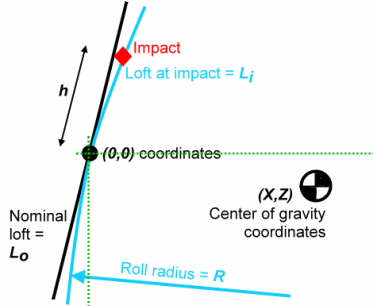First thing, we need a coordinate system so we can describe the center of gravity placement, the impact of ball on clubface, etc. Let's use a two-dimensional system with axes x (horizontal along the target line) and z (vertical). We will set the origin at the point on the clubface where the nominal loft is measured. If we talk about a 10.5º clubhead, then [0,0] is the point on the clubface where the loft is actually 10.5º. (Because of face roll, there is only one height on the clubface where the loft is exactly 10.5º.)

Within this coordinate system, we can begin to describe the parameters of our analysis.
• We designate nominal loft as Lo. The [0,0] point is where the black line at an angle of 90º-Lo is tangent to the blue clubface.
• The placement of the CG is at [X, Z]. X is a depth from the face, and Z is height above the nominal-loft height. A low CG (as in the diagram) corresponds to a negative Z.
• Impact occurs h above the nominal loft, measured along the clubface. If it occurs lower on the clubface than nominal loft, h is negative.
• The effective loft where impact occurs is Li. The diagram shows Li as incorporating the effect of face roll, but it will also include clubhead rotation due to shaft bend. The face roll is specified by a radius of R. In most driver heads, R is between 10 and 14 inches.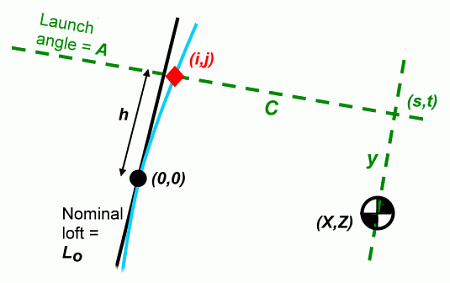Having established a coordinate system, we can use it to compute C and y, the quantities that will determine the spin. Our strategy will be to find the [x,z] coordinates of two points in space, [i,j] and [s,t]. Then:
• C is the distance between [i,j] and [s,t].
• y is the distance between [s,t] and [X,Z].
Let's go through the steps.

First compute Li.

Li  =  Lo + arcsin (h/R) + 1.5 (X-F)

The arcsin(h/R) accounts for face roll for impact h inches above nominal. The "1.5" factor accounts for loft change due to shaft bend, explained later on this page.

Once we have the effective loft, we use the "standard" formula to get the launch angle.

A  =  Li (0.96 - .0071 Li)

We can also get good values for [i,j].

i  =  h sin (Lo + 1.5(X-F)) + h2/2R
j  =  h cos (Lo + 1.5(X-F))

In each case, the sine-cosine factor accounts for position along the black line. The h2 factor for i accounts for the small space between the black line and the blue curve at impact. (We aren't going to worry about the tiny difference in j due to the launch angle.)

Now let's get values for [s,t]. This is a little harder, but we can use the fact that C is measured along the launch line and y is perpendicular to the launch line. Let's start by defining the slope of the launch angle as m, then use the point-slope method (remember, back in high school algebra?) to define the lines of C and y. This is why we want a coordinate system; we can treat the whole diagram like a graph.

m  =  tan (A)

Now we can write the equations for the two green lines. The equations are easily found using the point-slope form. The line for C (along the launch line) goes through the point [i,j] at slope -m. The line for y goes through the point [X,Z] at slope 1/m (the perpendicular to -m). So the lines in point-slope form are:

z - j  =  -m (x - i)
z - Z  =  1/m (x - X)

Next we need to find the point [s,t]. We know that the two green lines intersect at [s,t]. So let's solve the two equations for x and z, and set those values to s and t. The solution is:

s  =  (m / (m2+1)) ( j + mi - Z + X/m )
t  =  m (i-s)  +  j

So now we know the coordinates of the points [i,j], [s,t], and [X,Z]. What we really want is C and y, but those are just distances between the points. We find those distances with the Pythagorean Theorem.

C2  =  (s-i)2 + (t-j)2
y2   =  (s-X)2 + (t-Z)2

However, y can be negative as well as positive. The Pythagorean Theorem gives the magnitude but not the sign. So let's use trigonometry instead.

y   =  (t-Z) / cos(A)

Now we have everything we need to find the spins, using formulas from basic impact and gear effect.

Vball  =  Vclub * SmashFactor * cos (Li)

SPINGE  =  58830 * Vball * C * y / Iv

SPINloft  =  160 * Vclub * sin (Li)

SPIN  =  SPINloft  -  SPINGE

This is very close to the calculations in the spreadsheet. (We took a few shortcuts and consolidations in the spreadsheet.)

Calculations for launch angle

(This is the weakest rationale for any of the calculations in this article. I assert here that the mechanism at work is the change in loft due to the rotation of the clubhead during impact. If there is some other mechanism at work, the conclusions here may be wrong.)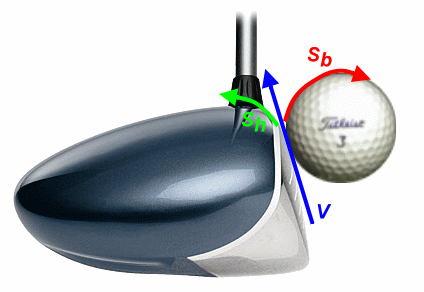One factor that changes launch angle is the head rotation due to gear effect. The head is the big gear that drives the little gear -- the ball. So the head will spin at a rate proportional to the ball's spin rate (just the gear effect spin) and also proportional to the "gear ratio", the ratio of diameters. Expressed as an equation:

What are these equal to?
• BallSpin: we computed that already as SGE. We'll use the number we computed.
• BallRadius: 0.84 inches, controlled by the Rules of Golf. (Actually, the Rules controls the diameter at 1.68 inches.)
• HeadSpin: what we want to find.
• HeadRadius: by definition, the distance from the center of rotation (the CG) to the face. We recognize it as the computed value of C.
So the equation becomes:

 HeadSpin  Sh  =  0.84 SGE C

The unit is RPM. We're looking for loft change, and we measure loft in degrees. Actually, degrees per second would be a much more convenient unit for HeadSpin. So we want to multiply by 360 (to get to degrees) and divide by 60 (to get to seconds). The equation becomes:

 Sh  = 0.84 * 360 60 SGE C =  5.04 SGE C

Sh is a spin rate, an angular velocity, measured in degrees per second. But what we really want is a total rotation in degrees. That is the amount that the loft can be considered to change, thus changing the launch angle. Just as distance is the accumulation of velocity ("integration" in calculus terms), the rotation is the accumulation of angular velocity. So we need to know the angular velocity function during impact, and integrate it to get the rotation of the clubhead.

The angular velocity does not jump to Sh from the beginning of impact, just as ball spin doesn't jump instantly to SGE. Let's assume it climbs on a straight line. This is a plausible approximation, though it is more likely a slight S-curve; that is, the angular acceleration on the gear pair -- the clubhead and ball -- probably builds as the ball gradually compresses and falls off as the ball releases. But let's keep things simple and assume a straight-line increase.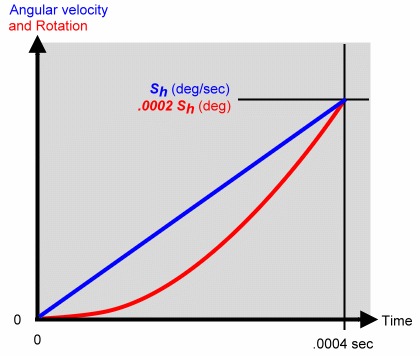The graph on the right shows the angular velocity in blue, as it increases from zero at the beginning of impact to Sh when the ball releases .0004 seconds later. The equation for the straight-line angular velocity function is:

 ω  = Sh t .0004

In order to find the angle of rotation at any time T after the beginning of impact, we have to integrate the angular velocity.

 θ(T) = T ∫ t=0 Sh .0004 t dt = Sh .0008 T2

The function for angle of rotation θ(T) is the red curve. We can see from the equation and the graph that it is a square-law parabola. At the moment of separation (T=.0004 sec), the clubhead has rotated .0002Sh degrees.

But is that what we really want? The loft as the ball leaves is not likely to be the angle that most affected the launch angle; the effective loft is probably somewhere in the middle of impact, e.g. the point of maximum compression of the ball on the clubface. So what is the clubhead rotation in the middle of impact? That is T=.0002 seconds. We evaluate it as:

 θ(T=.0002)  = Sh .0008 (.0002)2  =  .00005 Sh

This is only a quarter of the rotation we found for separation. Not a half, but a quarter. If you're familiar with quadratic functions -- or even take a good look at the red curve in the graph -- this is not surprising.

We will use this value as the loft change due to gear effect, as we estimate the change in launch angle. If you want to see what a higher fraction of the head rotation would do, the spreadsheet allows the fraction as a parameter; the default is 0.25, but you can adjust it.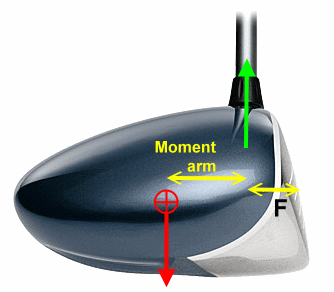Centrifugal force pulling on the clubhead's CG creates a turning moment, trying to rotate the face upwards. The moment is equal to the magnitude of the centrifugal force times the "moment arm", the distance between the centerline of the shaft and the clubhead's center of gravity. This moment is resisted by the stiffness of the shaft. So how do we factor in all these parameters?

Jeff Summitt has given me a rule of thumb that he says works over a variety of clubheads and shafts. The clubhead rotates to increase the loft by 0.15º for each 0.1 inch increase in the moment arm. That is a rate of 1.5º per inch, though Jeff is quick to remind me that moving the CG by an inch is huge. Yes, that rate varies a bit with the velocity, clubhead mass, and shaft stiffness, but the difference is small enough that you can ignore the variation.

LoftChange  =  1.5º * MomentArm
• LoftChange in degrees
• MomentArm in inches
• v in miles per hour
If we want more resolution than this, the formula has to account for:
• Velocity, which is a square-law factor in centrifugal force.
• Radius of the clubhead's path at the moment of impact. That is typically somewhat more than the club length -- maybe 25% more for a full swing with good release.
• Bending properties of the shaft. Not just the overall stiffness, but the flex profile over the length of the shaft. This can be characterized by an EI profile, a frequency profile, or a deflection profile -- and each has its own computational difficulties. The EI profile is probably the most elementary flex profile for use in a computation, but is rather rare to have around for a given shaft. (Russ Ryden has been characterizing shafts by EI profile.)

So there you have it, a set of calculations embodied in a spreadsheet, to compute the effect of CG placement on spin and launch angle.

Last updated - Mar 10, 2014